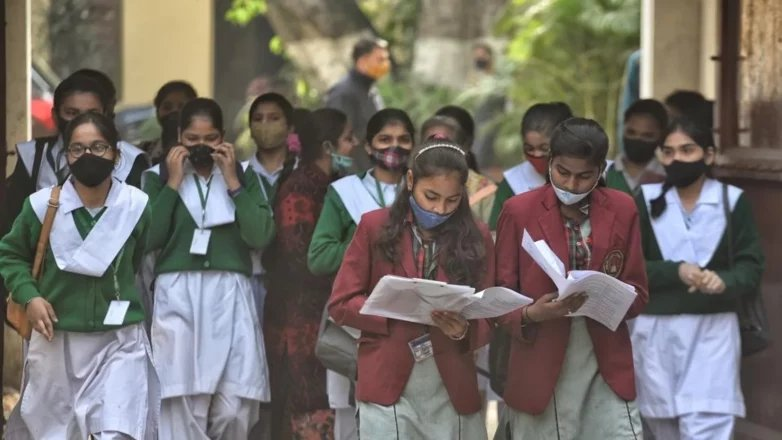By | 23 Sep 2022 at 11:16 PMCBSE Class 10 Mathematics (Basic) Sample Question Paper 2022-23: The Central Board of Secondary Education has released the Class 10 Maths (Basic) Sample Paper for 2022-23. Subject wise sample papers along with their marking scheme are available on the CBSE website.

Practice is the most important thing during the preparation of maths. By preparing with the help of sample paper, students can get more marks in the exam.

If you are afraid of big syllabus and don’t know where to start, then you can start with NCERT exercises. Once you complete the NCERT Mathematics Syllabus, then solve CBSE Class 10 Maths (Basic) Sample Question Papers 2022-23, and other previous year papers regularly. Doing this will help them maintain all the formula and will also help with your speed and confidence.

Click here to get the NCERT textbook pdf for CBSE Class 10 Mathematics

Many students make the mistake of solving sample papers without even practicing NCERT exercises, which only gives them confidence and nervousness. In such a situation, it is necessary to do NCERT exercises. This will make you basic strong.

Some instructions are given in CBSE Class 10 Maths (Based) Sample Question Paper 2022-23. Read here in detail.

There are 5 sections A, B, C, D and E in this question paper.

Section A consists of 20 Multiple Choice Questions (MCQs) carrying 1 mark each.

– Section B consists of 5 Short Answer-I (SA-I) type questions carrying 2 marks each.

– Section C consists of 6 Short Answer-II (SA-II) type questions carrying 3 marks each.

Students can check below the complete contents of CBSE Class 10 Maths (Basic) Sample Question Paper 2022-23 and prepare for Maths (Basic) Board Exam 2022-23.

CBSE Sample Paper 2023: Check Here 10th-12th Sample Paper

The Central Board of Secondary Education (CBSE) has released the sample question paper for class 10th and 12th 2022-23. This time only one test will be taken. In addition to practical exam, there will be theory exam. According to the board, there will be questions in five sections in class X. The first section will be of objective type questions. This time 20 questions of one mark each will be asked. The examination of science subject in class 10th will be of 70 marks. There will be 39 questions in it. At the same time, there will be an examination of 80 marks for social science. 37 questions will be asked in it.

CBSE Class 10 sample question paper for 2022-23

CBSE Class 12 sample question paper for 2022-23